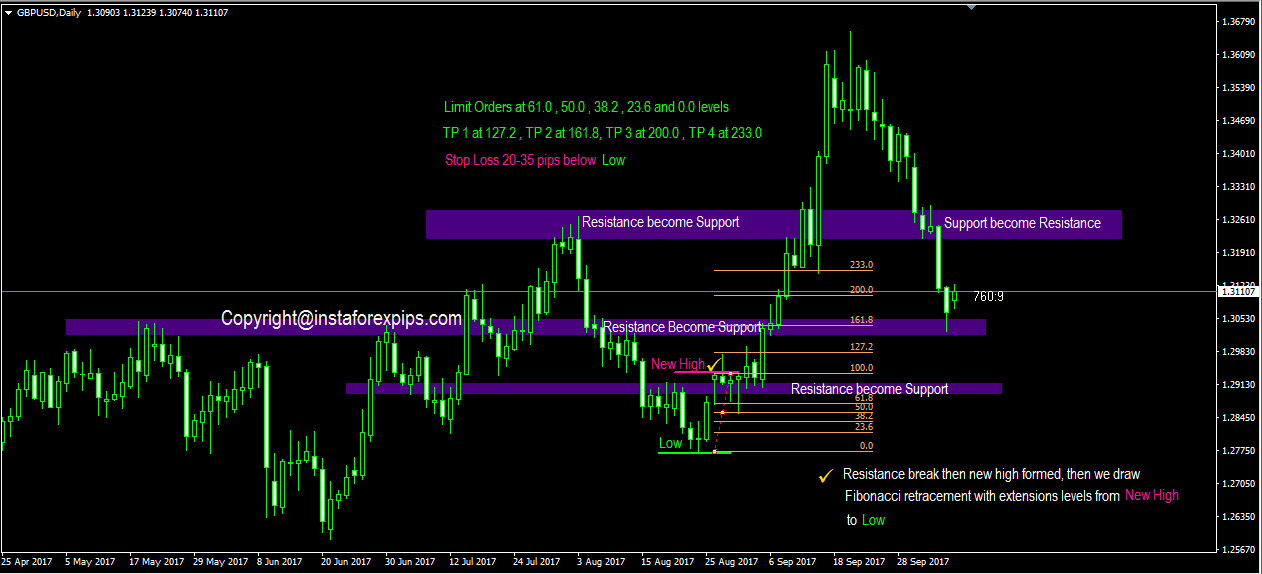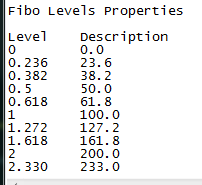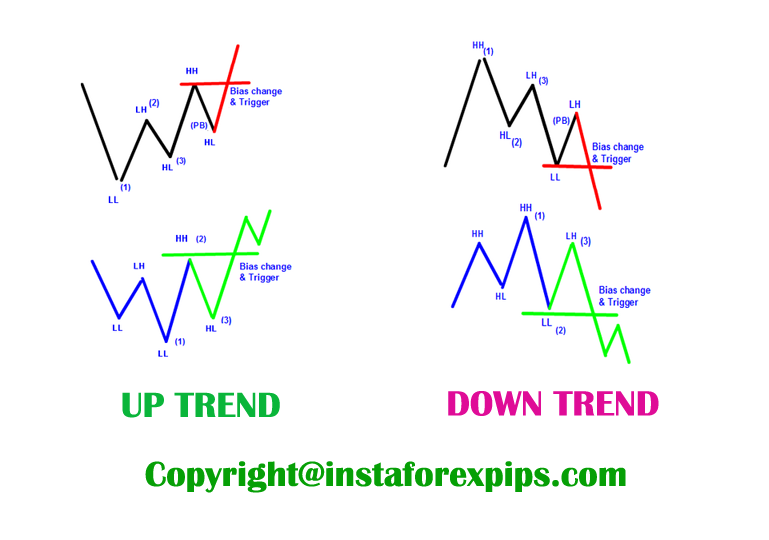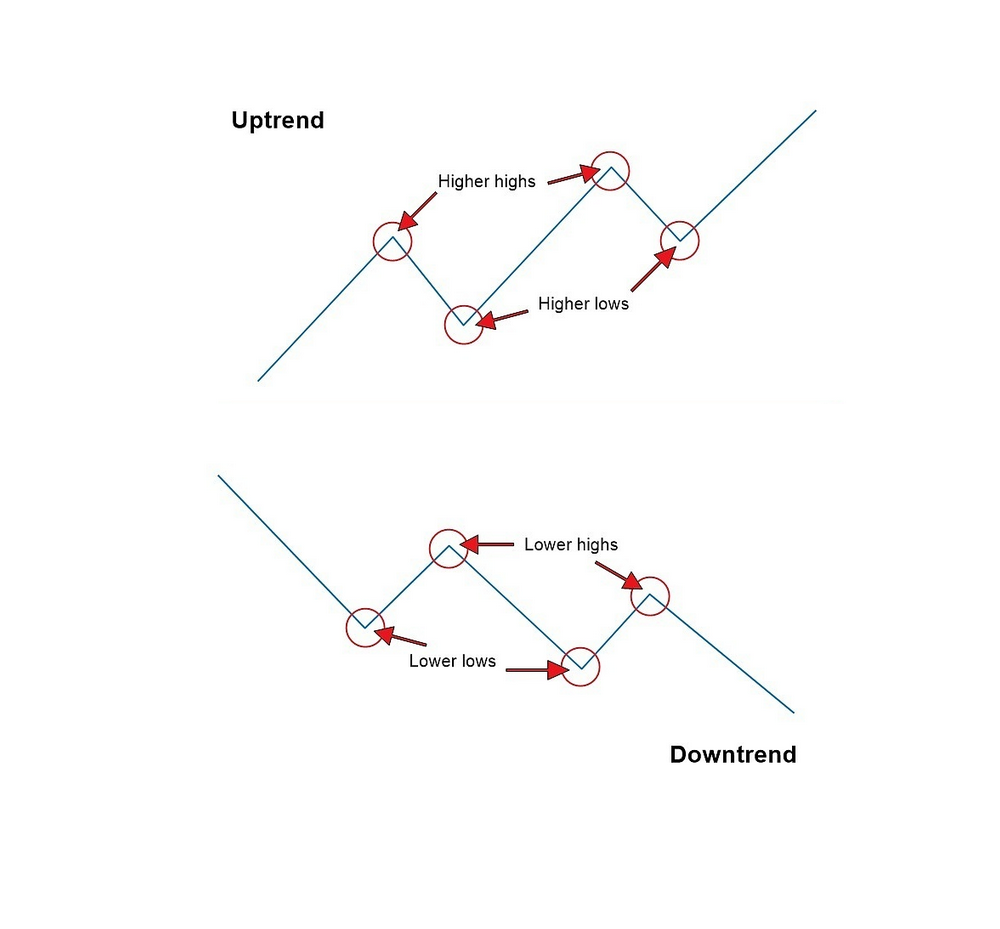## Calculate Entry Point Level With Fibonacci Retracement## Trading With Fibonacci Retracement Strategy

Trading forex with fibonacci retracement is very help full to calculate range before we make decision to open position. Basically fibonacci retracement is very important to calculate price level to retrace levels which have high probabilities price levels to bounce the price in order to continue price movement from the main trend. Fibonacci retracement strategy need combined with break out forex support resistance strategy then we draw fibonacci retracement from a new fresh low or new fresh high to get retracement level to placed limit orders and adding some fibonacci extensions projection levels as our take profit levels. And also we can add reversal candlestick patterns as our confirmation trading signals. The main point in how we use fibonacci levels are we need to wait first support resistance breakout then we draw fibonacci with opposite direction then wait the candlestick reversal patterns confirmed as our signals entry. The golden ratio from fibonacci are 38.2 , 50,0 and 61.8. So, on those fibonacci levels there’s high chance to bounce the price. And stop loss can be placed a few pips from 0.00 level of fibonacci ratios. More over we need adding some fibonacci extensions as our swing projection that will be as take profit levels.

## Fibonacci History That We Need To Know

A few hundreds years ago, an italian mathematician described that nature and numbers have a strong correlation. His name is Fibonacci. He introduced an interesting number sequence starting from zero and one (0, 1).

Take a look below, as we build a Fibonacci sequence:

0, 1

Now we perform the following calculation, where we add to the last number to the previous number in the sequence:

0 + 1 = 1

The result we get here (one) is the next number in the sequence. Thus, we now have the following:

0, 1, 1

Now we add the last number in the sequence to the previous number as shown below:

1 + 1 = 2

Then we add 2 to our sequence:

0, 1, 1, 2

We repeat the process of adding the last number in the sequence to the previous number:

1 + 2 = 3

…and the next one:

0, 1, 1, 2, 3

……and the next one:

0, 1, 1, 2, 3, 5

……….and the next one:

0, 1, 1, 2, 3, 5, 8

………….and the next one:

0, 1, 1, 2, 3, 5, 8, 13

We repeat this process as many times as we want!

Now you know how fibonacci derived the numbers – 0, 1, 1, 2, 3, 5, 8, 13, 21, 34, 55, 89, 144, 233, 377.

Key Fibonacci Ratios

But what is so interesting about these numbers? As you probably guess, the fun part begins now!

So, Fibonacci discovered the following: every number of this infinite sequence is approximately 61.8% of the next number in the sequence. If you do not believe me, let’s dive into the math for ourselves!

55 / 89 = 0.6179775280898876 = 61.8%

Wow! Let’s double check!

233 / 377 = 0.6180371352785146 = 61.8%

Do you believe me now? No? Ok then:

144 / 233 = 0.6180257510729614 = 61.8%

I think this is persuasive enough!

However, this is not the only thing, which Fibonacci introduced. He also found out that every number in this sequence is approximately 38.2% of the number after the next one in the sequence:

13 / 34 = 0.3823529411764706 = 38.2%

21 / 55 = 0.3818181818181818 = 38.2%

55 / 144 = 0.3819444444444444 = 38.2%

144 / 377 = 0.3819628647214854 = 38.2%

And also, we have another ratio! Every number in the Fibonacci sequence is 23.6% of the number after the next two numbers in the sequence:

55 / 233 = 0.2360515021459227 = 23.6%

Note that these percentages can be retrieved from each number pair, which is part of the Fibonacci sequence, continuing to eternity.

Fibonacci Ratios Can Be Found Anywhere

So, this is how the famous 61.8%, 38.2% and 23.6% Fibonacci levels appear.

This is the 76.4% level which is 100 – 23.6. But, what is so important about these percentages? The truth is that these ratios are all over the place in nature. Look at this several pictures below :## How To Calculate Fibonacci Retracement Levels As Our Trading Levels Based Support Resistance Strategy

As a described from first paragraph, how to get a accurate trading signals based fibonacci trading principles, i make a simple trading using fibonacci trading strategy from daily chart in gbpusd based breakout support resistance confirmation.

Let’s take a look a picture below : 😉From picture of trading scenario above, we can see nearest resistance of 1.29148 already breakout and make a new high or higher high it means trend up just happened. Then draw fibonacci retracement from new higher high to lower low and adding fibonacci extensions levels as described from picture below.After draw fibonacci retracement which adding fibonacci extensions levels then we can placed pending limit orders on 61.8,50.0,38.2,23.6 and we hope on these levels there’s a reversal candlestick patterns appear. Reversal candlestick patterns or wick as our rejection price can be seen in current time frame or lower time frame. So, checked all time frame to find reversal candlestick patterns confirmation as our best trading signals. Once again wait until this reversal candlestick appear first. Stop loss can be placed several pips below 0.0 level. And for take profit level 1 at 127.2 , take profit level 2 at 161.8 , take profit level 3 at 200.0 , take profit level 4 at 233.0 .

The basic principle to draw fibonacci retracement are very simple, just follow the basics principle of price action trading strategy to determine a market’s trend. If there’s a first higher low then price breakout previous lower high and making first higher high so trend up setups it’s just happened and if there’s a first lower high then price breakout previous higher low and making first lower low so it means trend down it’s just happened. See pictures below to learn how simple price action trading setups help us in determine the current main trend. Because we trade only follow the major trend right ? trend is our friend right ?.After we can identify current main trend with price action trading analysis, so at least from now on we can more confident to draw fibonacci retracement to get high probability levels to bounce the price and can predict projection price in making swing low or swing high.

In my opinion using daily chart it much better to start analyze current major trend before making any trading decisions. At least after read price action trading setup from daily time frame we can follow the trend or counter the trend. Once again fibonacci only second parameter to analyze where price to go or where price to retrace, so not for primary indicator to analysis. The first parameter is using price action trading strategy to read what main trend are running then combined it with major candlestick reversal which appear on golden ratios of fibonacci levels in current time frame or lower time frame.

Lastly, practice on daily first then looking best signals entry on four hour time frame, because daily are more strong or non lagging analysis which used as our first indicator start trading forex. With fibonacci strategy method we can calculating price with maximum swing and getting reversal entry levels to minimize our risk of trading.

Professional Forex Brokers That Have Been Tested And Recommended To Trading Forex :